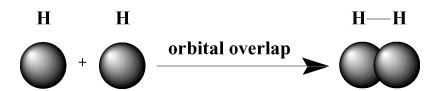# Valence bond Theory

Valence bond theory (VB) is a straight forward extension of Lewis structures. Valence bond theory says that electrons in a covalent bond reside in a region that is the overlap of individual atomic orbitals.

For example, the covalent bond in molecular hydrogen can be thought of as result of the overlap of two hydrogen 1 s orbitals.### Molecular Geometry

In order to understand the limitations of valence bond theory, first we must digress to discuss molecular geometry, which is the spatial arrangement of covalent bonds around an atom. A very simple and intuitive approach, the Valence Shell Electron Pair Repulsion (VSEPR) model, is used to explain molecular geometry. VSEPR states that electron pairs tend to arrange themselves around an atom in such a way that repulsions between pairs are minimized./PARGRAPH For instance, VSEPR predicts that carbon, which has a valence of four, should have a tetrahedral geometry. This is the observed geometry of methane ( CH 4 ). In such an arrangement, each bond about carbon points to the vertices of an imaginary tetrahedron, with bond angles of 109.5 degrees, which is the largest bond angle that can be attained between all four bonding pairs at once. Similarly, the best arrangement for three electron pairs is atrigonal planar geometry with bond angles of 120 degrees. The best arrangement for two pairs is a linear geometry with a bond angle of 180 degrees.

### Valence bond theory limitations

1. explains, but does not predict

2. qualitative explanations; does not explain relative stability

3. can’t explain color and spectra

4. can’t explain relative stability of structural isomers

### drawbacks of valence bond theory

1.it does not explain the shape and geometry

2.it does not explain the tetravalency of carbon